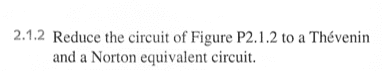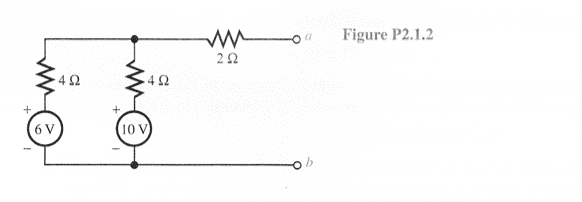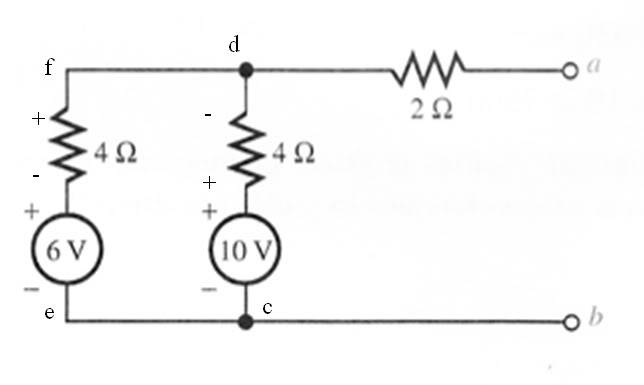# Circuits: Thevenin Equivalent Circuit

## Homework Statement## The Attempt at a Solution

I am not getting this at all. I know that I need to calculate the open circuit voltage Voc and the short circuit current Isc. I really have no idea how to find Voc. There is some conceptual leap I need to make here. When the circuit is "open" across a-b, I know that no current flow through the 2 ohm resistor and this allows me to find the current flowing through the left loop (which is = 0.5 A ). But I have no idea how that helps me to find Voc = Vab which is outside of that loop.

Homework Helper
You know the current so you can calculate the potential drop across the 4 ohm resistor connected in series with the 10 V battery. With respect to b, the potential is 10 V at the upper terminal of the battery. The current flows upward, so the potential drops across the 4 ohm resistor, but there is no change of potential across the 2 ohm resistor. So what is the potential at b?

You know the current so you can calculate the potential drop across the 4 ohm resistor connected in series with the 10 V battery. With respect to b, the potential is 10 V at the upper terminal of the battery. The current flows upward, so the potential drops across the 4 ohm resistor, but there is no change of potential across the 2 ohm resistor. So what is the potential at b?

Hello ehildI am confused as to why we do not consider the outer loop with the 6 V battery?

Homework Helper
You did consider it when you calculated the current.
The potential at a can be calculated from any path between a and b. If you take the path that contains the 6 V battery, you have to add the voltage across the resistor as the current flows downward.

ehild

Hi again ehild. Thank you for you patience, this is not very intuitive for me. I have attached a new diagram in which I have drawn in the polarities and added some labels. Please tell me if this is correct:1) The open circuit voltage is measured across ports a and b, which means I let b be my zero reference voltage and I measure the voltage at a with respect to b (or I could work from a to b).

2) To measure the voltage, I can step through either branch of the circuit: bcda or befa and algebraically sum the voltages.

So, for branch bcda we have: - 10V + 4Ω*0.5A = - 8V
And for branch befa we have: - 6V - 4Ω*0.5A = -8V

Is this good so far? If so, can we talk about the meaning of the signs? If we instead measured from a to b, we would have gotten positive 8V. Does this just tell me the direction of the current at the load resistor?

Mentor
Going from c to d you're going UP 10V then DOWN I*4Ω = 2V . So the voltage at a should be positive 8V.

Going from c to d you're going UP 10V then DOWN I*4Ω = 2V . So the voltage at a should be positive 8V.

Hi gneillWe have always gone with the following convention: As you pass through the loop and encounter an element, you assign it the first sign that you encounter. So as I go up through the 10V source, I encounter its (-) terminal first and hence assign it a (-) value. I guess it makes more sense to do the opposite since it gets you a positive value for a source.

But anyway, more importantly, my procedure is correct? I can use either branch to find Voc?

Now I also need to find the short circuit current Isc. I do this by "attaching" a wire from a to b and finding the equivalent current in the circuit. So I know how to combine resistances when there is a simple circuit with a single source and a bunch of resistors, but I am not so sure as to what to do when there are multiple sources? I feel like I need to combine them, but I am not sure how? Any suggestions on this?

Last edited:
Mentor
Yes, you can use either branch to find the resulting output voltage.

If you are having trouble determining the short circuit current, then I can suggest a few ways to proceed. First, you could just use KVL loops for the two loops and solve for the current. Second, you could find the Thevenin equivalent of the circuit before the 2 Ohm resistor first; for that step you'd have a direct short across the legs and you'd just have the currents caused by each source through its own series resistor to sum up. Add the 2 Ohm resistor back in afterward; you'll have a single supply in series with its Thevenin resistance and the 2 Ohm resistor. Third, you could convert each source and resistor into its Norton equivalent to begin with, then combine into a single Norton equivalent. The short circuit current would then be given by the current through the 2 Ohm resistor as a parallel branch (Norton current splitting between the Norton resistance and the 2 Ohm resistance).

Yes, you can use either branch to find the resulting output voltage.

If you are having trouble determining the short circuit current, then I can suggest a few ways to proceed. First, you could just use KVL loops for the two loops and solve for the current. Second, you could find the Thevenin equivalent of the circuit before the 2 Ohm resistor first; for that step you'd have a direct short across the legs and you'd just have the currents caused by each source through its own series resistor to sum up. Add the 2 Ohm resistor back in afterward; you'll have a single supply in series with its Thevenin resistance and the 2 Ohm resistor. Third, you could convert each source and resistor into its Norton equivalent to begin with, then combine into a single Norton equivalent. The short circuit current would then be given by the current through the 2 Ohm resistor as a parallel branch (Norton current splitting between the Norton resistance and the 2 Ohm resistance).

Ok thenI will use the first method since I am in the process of learning about Thevenin and Norton equivalents and will hopefully reduce this circuit to both. But for now, I will use KVL (using my sign convention) :

KVL bcdab:
-10V + icd*4Ω + ida*2Ω = 0

KVL befa:
-6V - ief*4Ω + ida*2Ω = 0

And I am going to take a wild guess here that I need to use KCL to eliminate the 3rd unknown current.

KCL Node d:
ida=ief + icd

So I can each of the individual currents here, but how do I get Isc from this information?

Mentor
So I can each of the individual currents here, but how do I get Isc from this information?

Ok! So Isc is simply the current that flows through the "wire" that I attched in order to short the circuit!

So my Thevenin Equivalent circuit is simply a voltage source with a value of VTH = Voc that is in series with a resistor with a value of RTH = VTH/Ioc.

Correct? If so, I would like to move on to the Norton equivalent. All I need to do is use the fact that:

RTH = RNo
VTH = INoRNo.

So we have that

INo = VTH/RNo = VTH/RTH

and it looks from all this that my short circuit current is the norton equivalent current Isc.

So really, there is no additional work required to find the Norton equivalent? I simply replace the circuit with a current source whose value is INo=Isc that is in parallel with a resistor whose value is RNo = RTH.

Correct?

Homework Helper
Correct.

ehild

Correct.

ehild

Great!Those conceptual leaps I spoke of are occurring as we speak! Now, I would like to ask a few more questions if anyone has the time and patience to discuss with me.

1) Why are the Thevenin & Norton equivalents the way that they are? That is why is the former a Voltage source in series with resistor and the latter is a current source in parallel with the same resistor? My text does not cover this and I am currently looking at some online resources, but they are incomplete. They mention that Kirchoff's Laws, Superposition and Ohm's Law are the key, but that's where it stops.

That's all for now ...Mentor
Well, imagine what would happen to an ideal current source connected to a series resistor and nothing else (like it was sitting in inventory and not connected to anything!). The voltage would go to infinity as it tried to drive its required current through an open circuit. Kablooey! With the resistor across it, it just sends its current through that and produces the open circuit voltage that you expect (the Thevenin voltage!).

Imagine what would happen to an ideal voltage source with a permanent resistor across it. No problem, right? It'll always produce its allotted voltage. But what happens when the output is shorted? Bang! Infinite current. That's not what the original circuit would do. When shorted it should produce the short-circuit current of the original circuit. That is, the Norton current!

So the two models are very closely related. The resistance is the same for both, and they both produce identical short and open circuit behavior.

Sounds good to me! I think! I feel like the Norton equivalent might be helpful in my other thread about conservation of power. I am going to head over there now and post what I am thinking!

Thanks again gneill and ehild !!!

Mentor
Since the originating poster has solved the problem, there should be no worries about demonstrating another method to solution.

In the attached image, the circuit is analyzed by successive Norton and Thevenin transformations that allow stepwise simplifications to be made.

#### Attachments

• Thev1.pdf
24.6 KB · Views: 398
Ok thenI will use the first method since I am in the process of learning about Thevenin and Norton equivalents and will hopefully reduce this circuit to both. But for now, I will use KVL (using my sign convention) :

KVL bcdab:
-10V + icd*4Ω + ida*2Ω = 0

KVL befa:
-6V - ief*4Ω + ida*2Ω = 0

And I am going to take a wild guess here that I need to use KCL to eliminate the 3rd unknown current.

KCL Node d:
ida=ief + icd

So I can each of the individual currents here, but how do I get Isc from this information?

Hmmm ... something horrible is happening here. When I solve the three equations above to find ida = isc I am getting 1A. Double checked (each a different way of solving the system).

If I use this value, I get RTh = 8/1 = 8Ω which I know is not the right answer.Mentor
Must be a math error somewhere. I just solved your equations and arrived at 2A.

Must be a math error somewhere. I just solved your equations and arrived at 2A.

Hmmm. I just went through it again, and got 1A. I am going to go through it here and hopefully I will catch my error while typing. Using the equations:

...

-10V + icd*4Ω + ida*2Ω = 0 (1)

-6V - ief*4Ω + ida*2Ω = 0 (2)

ida=ief + icd (3)

From (3) → icd = ida - ief

Plugging this result into (1):

-10 + 4ida - 4ief + 2ida = 0
→ -10 + 6ida - 4ief = 0

Multiply this result by -1 to yield:
10 - 6ida + 4ief = 0

10 - 6ida + 4ief = 0
-6 + 2ida - 4ief = 0
-------------------------------------------------------------
→ 4 + -4ida = 0
→ ida = 1A.Mentor
In your original equation (2), you've made an assumption about current direction (and hence voltage drops/rises) that doesn't jive with the other equations. If you really do have a voltage rise across the 4Ω resistor in the ef leg, then that same current should also produce a rise in the 2Ω resistor. Further, this makes the current direction for ida the opposite of that supposed for the other loop (bcda), and invalidating equation (3).

I find that the best way to write equations is to just assume one direction for all currents (clockwise, say), then all resistors in the path cause drops (are negative), and you take the voltage supply contributions to be positive if the current goes from - to + through them. If it so happens that the choice of direction for a given current was "wrong", then it'll come out negative in the end. No problem.

In your original equation (2), you've made an assumption about current direction (and hence voltage drops/rises) that doesn't jive with the other equations. If you really do have a voltage rise across the 4Ω resistor in the ef leg, then that same current should also produce a rise in the 2Ω resistor. Further, this makes the current direction for ida the opposite of that supposed for the other loop (bcda), and invalidating equation (3).

I find that the best way to write equations is to just assume one direction for all currents (clockwise, say), then all resistors in the path cause drops (are negative), and you take the voltage supply contributions to be positive if the current goes from - to + through them. If it so happens that the choice of direction for a given current was "wrong", then it'll come out negative in the end. No problem.

Ok. I will give this a try. You have been a tremendous help! I had another problem where I had to find the Thevenin and Norton equivalent circuits and instead of all this nonsense with KCL and KVL I just did piecewise Thevenin and Norton equivalents until I reached a circuit that had a single voltage source in series with a resistor. Took less than 2 minutes this way!

Homework Helper
Imagine you have a box with some network inside. If the network is linear, you get a linear relationship between output voltage and current by connecting different loads to the terminals:
aU+bI=1. If there is no load I=0 and the voltage is Uoc. If the output is shorted U=0 and the current is Isc. So the relation between U and I can be written in the form
U/Uoc+I/Isc=1.

Assume a box with the same U-I characteristics contains a voltage source with emf ε and inner resistance r in series. Then Uoc=ε and I/Isc=ε/r.
If the box contains a current source Is and a resistor r' parallel,
I/Isc=Is and Uoc//Isc=r'

Measuring Uoc and Isc, you get the emf and inner resistance of the Thevenin equivalent voltage source: ε=Uocand r=Uoc / Isc,
or the source current Is =Isc and internal resistance of the Norton equivalent current source: r'=Uoc / Isc=r. It is the same internal resistance in both equivalent circuits.

The internal resistance can also be obtained by calculating the resultant between the terminals if you replace all the voltage sources by shorts and removing the current sources in the original circuit.

ehild